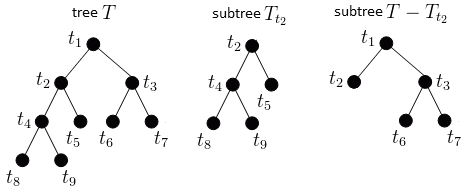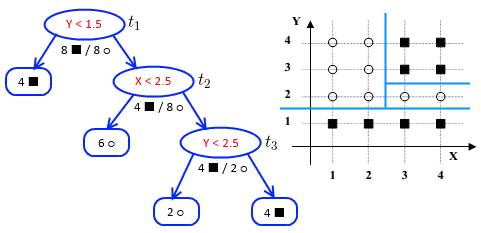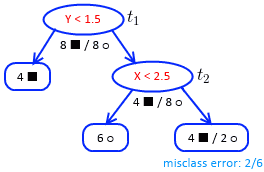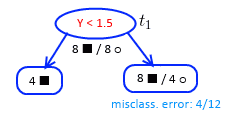ML Wiki

Cost-Complexity Pruning

Post-pruning algorithm for Decision Trees

• by Breiman, Olshen, Stone (1984)

Cost-Complexity Function

• need to optimize the cost-complexity function
• $R_\alpha (T) = R(T) + \alpha \cdot | f(T) |$ where
• $R(T)$ is the training/learning error
• $f(T)$ a function that returns the set of leaves of tree $T$
• $\alpha$ is a Regularization parameter
• $R(T) = \sum_{t \in f(T)} r(t) \cdot p(t) = \sum_{t \in f(T)} R(t)$
• $\sum_{t \in f(T)} R(t)$ - sum of misclassification errors at each leaf
• $r(t) = 1 - \max_k p(C_k - t)$ - misclassification rate
• $p(t) = \cfrac{n(t)}{n}$ with $n(t)$ being the # of records in node $t$ and $n$ - total # of records

Pruning Subtrees

Subtrees:

•Pruning a subtree $T_{t}$

• $R_\alpha(T - T_t) - R_\alpha(T)$ - variation of the cost-complexity function
• this is the cont-complexity when pruning subtree $T_t$
• $R_\alpha(T - T_t) - R_\alpha(T) = R(T - T_t) - R(T) + \alpha ( | f(T - T_t) | - |f(T)| ) = R(t) - R(T_t) + \alpha ( 1 - |f(T_t)| )$
• let $\alpha' = \cfrac{R(t) - R(T_t)}{|f(T_t)| - 1}$
• variation is
• null if $\alpha = \alpha'$
• negative if $\alpha < \alpha'$
• positive if $\alpha > \alpha'$

Algorithm

Pruning Algorithm:

• Initialization:
• let $T^1$ be the tree obtained with $\alpha^1 = 0$
• by minimizing $R(T)$
• Step 1
• select node $t \in T^1$ that minimizes
• $g_1(t) = \cfrac{R(t) - R(T^1_t)}{|f(T^1_t)| - 1}$
• let $t_1$ be this node
• let $\alpha^2 = g_1(t_1)$ and $T^2 = T^1 - T^1_{t_1}$
• step $i$
• select node $t \in T^i$ that minimizes
• $g_i(t) = \cfrac{R(t) - R(T^i_t)}{|f(T^i_t)| - 1}$
• let $t_i$ be this node
• let $\alpha^{i + 1} = g_i(t_i)$ and $T^{i+1} = T^i - T^i_{t_i}$

Output:

• a sequence of trees $T^1 \supseteq T^2 \supseteq \ ... \ \supseteq T^k \supseteq \ ... \ \supseteq \{ \text{root} \}$
• a sequence of parameters $\alpha^1 \leqslant \alpha^2 \leqslant \ ... \ \leqslant \alpha^k \leqslant \ ...$

Choosing $\alpha$

The algorithm outputs $\alpha^1 \leqslant \alpha^2 \leqslant \ ... \ \leqslant \alpha^k \leqslant \ ...$

• need to choose some $\alpha \in [\alpha^k, \alpha^{k+1} )$
• let $\alpha \in [\alpha^k, \alpha^{k+1} )$

How to choose $\alpha$

Example

Example 1

Suppose we have the following tree:

•• we want to prune it
• we have 3 inner nodes where we can prune: $t_1 \equiv \text{root}, t_2, t_3$

Some formulas:

• $R(T_t)$ - training error of a subtree $T_t$ - a tree with root at node $t$
• $R(T_t) = \sum_{l \in f(T_t)} R(l)$ - sum of all training errors over all leaves
• $R_(t)$ - training error of node $t$
• $R_(t) = r(t) \cdot p(t)$
• $r(t)$ - misclassification error at this none (without considering the leaves)
• $p(t)$ - proportion of data items reached $t$ (i.e. # of items reached $t$ divided by # of training items)
• $g(t) = \cfrac{R(t) - R(T_{T_t})}{| f(T_t) | - 1}$
• $| f(T_t) | - 1$ is the number of leaves to prune

Iteration 1:

• let $\alpha^{(1)} = 0$
$t$ $R_(t)$ $R(T_t)$ $g(t)$
$t_1$ $\cfrac{8}{16} \cdot \cfrac{16}{16}$ $T_{t_1}$ - the entire tree
all leaves are pure
$R(T_{t_1}) = 0$
$\cfrac{8/16 - 0}{4 - 1} = \cfrac{1}{6}$
$t_2$ $\cfrac{4}{12} \cdot \cfrac{12}{16} = \cfrac{4}{16}$
(there are 12 records, 4 $\blacksquare$ + 8 $\bigcirc$ )
$R(T_{t_2}) = 0$ $\cfrac{4/16 - 0}{3 - 1} = \cfrac{1}{8}$
$t_3$ $\cfrac{2}{6} \cdot \cfrac{6}{16} = \cfrac{2}{16}$ $R(T_{t_3}) = 0$ $\cfrac{2/16 - 0}{3 - 1} = \cfrac{1}{8}$

We want to find the minimal $g(t)$

• it's $g(t_2)$ and $g(t_3)$
• in case of a tie, we choose the one that prunes fewer nodes
• i.e. $g(t_3)$
• so prune at $t_3$
• let $\alpha^{(2)} = 1/8$ (the min $g(t)$)Iteration 2:

• in the tree now we have only candidates: $t_1$ and $t_2$

$t$ $R_(t)$ $R(T_t)$ $g(t)$
$t_1$ $\cfrac{8}{16} \cdot \cfrac{16}{16}$ $\cfrac{2}{16}$ $\cfrac{8/16 - 2/16}{3 - 1} = \cfrac{6}{32}$
$t_2$ $\cfrac{4}{12} \cdot \cfrac{12}{16}$ $\cfrac{2}{16}$ $\cfrac{4/16 - 2/16}{2 - 1} = \cfrac{1}{8}$

Find minimal $g(t)$:

• it's $g(t_2) = 1/8$
• let $\alpha^{(3)} = 1/8$
• prune at $t_2$
•Iteration 3:

• only one candidate for pruning: $t_1$
• $\alpha^{(4)} = g(t_1) = \cfrac{8/16 - 4/16}{2 - 1} = \cfrac{1}{4}$

Selecting the best:

• we have these values: $\alpha^{(0)} = 0, \alpha^{(1)} = 1/8, \alpha^{(2)} = 1/8, \alpha^{(3)} = 1/4$
• by the theorem we want to find tree such $T$ that minimizes the cost-complexity function
• if $0 \geqslant \alpha < 1/8$, then $T_1$ is the best
• if $\alpha = 1/8$, then $T_2$ is the best
• if $1/8 < \alpha < 1/4$, then $T_3$ is the best
• if $1/8 < \alpha < 1/4$, then $T_3$ is the best
• to choose $\alpha$ use Cross-Validation

Example 2

From IT4BI 2013 year exam: